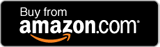Live Sandbox PHP decbin(), dechex() & decoct() Functions
PHP decbin(), dechex() & decoct() Functions
##### Instructions/Notes

decbin(integer) - This PHP function takes an integer (base-10/decimal) input and converts it to a binary (base-2) number. The largest number that can be converted is 4294967295 in decimal.

dechex(integer) - This PHP function takes an integer (base-10/decimal) input and converts it to a hexadecimal (base-16) number. The largest number that can be converted is 4294967295 in decimal resulting to "FFFFFFFF".

decoct(integer) - This PHP function takes an integer (base-10/decimal) input and converts it to an octal (base-8) number. The largest number that can be converted is 4294967295 in decimal resulting to "37777777777".

Convert the following number